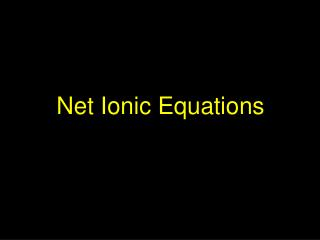DownloadDownload PresentationNet Ionic Equations

# Net Ionic Equations

Télécharger la présentation## Net Ionic Equations

- - - - - - - - - - - - - - - - - - - - - - - - - - - E N D - - - - - - - - - - - - - - - - - - - - - - - - - - -
##### Presentation Transcript

1. Net Ionic Equations

2. AgNO3 (aq) + NaCl(aq) AgCl(s) + NaNO3(aq) What happens when you put AgNO3 and NaCl in water? (both are ionic compounds)

3. KEY POINT: NaCl (aq) really means: Na +1(aq) + Cl -1(aq) “dissociated ions” or “ions in solution”

4. KEY POINT: 2Mg(NO3)2(aq) really means: 2Mg +2(aq) + (NO3) -1(aq) 4 “dissociated ions” or “ions in solution”

5. KEY POINT: AgCl (s) really means: AgCl (s) Solid Silver (I) Chloride.

6. What is Dissolving ? When an ionic compound (eg salt) dissolves in water, the compound disassociates. (breaks apart into cations and anions) Ex: NaCl(s)Na1+(aq) + Cl-(aq) H2O When a covalent compound (eg sugar) dissolves in water, the molecules simply disperse; they do not disassociate.

7. An Ionic Compound Dissolves: A Covalent Compound Dissolves:

8. AgNO3 (aq) + NaCl(aq) AgCl(s) + NaNO3(aq) What happens when you put AgNO3 and NaCl in water?

9. Initial addition of salts AgNO3 NaCl

10. NO3- Na+ Ag+ Cl-

11. Na+ NO3- Ag+ Cl-

12. AgCl precipitates begin to form Na+ NO3- AgCl

13. Na+ NO3- AgCl(s) precipitate AgCl

14. NO3- Na+ NO3- NO3- Na+ Na+ Na+ NO3- NO3- Na+ Na+ Na+ NO3- NO3- Na+ NO3- Na+ AgCl(s)

15. AgCl Na+ NO3- Reaction

16. No Reaction - remains as ions Na+ NO3- AgCl

17. These ions do not participate in the reaction. They are called SPECTATOR IONS Na+ NO3- AgCl

18. AgNO3 (aq) + NaCl(aq) AgCl(s) + NaNO3(aq) complete ionic equation

19. NO3- and Na+ appear on both sides of the equation (spectator ions - do not take part in the reaction)

20. net ionic equation

21. Example 2 Cd(NO3)2 (aq) + (NH4)2S(aq) CdS(s) + 2NH4NO3(aq) complete ionic equation Cd+2 (aq) + 2NO3-(aq) + 2NH4+ (aq)+S-2(aq) CdS(s) + 2NH4+(aq) + 2 NO3- (aq)

22. net ionic equation Cd+2 (aq) + 2NO3-(aq) + 2NH4+ (aq)+S-2(aq) CdS(s) + 2NH4+(aq) + 2 NO3- (aq) CdS(s) Cd+2 (aq) +S-2(aq)

23. Complete ionic equation- • More accurately shows the reacting species as ions and the products either as ions or a precipitate • Net ionic equation- • Focuses only on the ions reacting. • Spectator ions are those ions that do not participate in the reaction.

24. Basic Chemical equation: AgNO3(aq) + KCl(aq) AgCl(s) + KNO3(aq) Complete ionic equation shows ions in solution: Ag+ (aq) + NO3- (aq) + K+ (aq)+ Cl-(aq) AgCl(s) + K+ (aq) + NO3-(aq) Net ionic equation shows ions in rxn: Ag+ (aq) + Cl-(aq) AgCl(s) (leave out spectator ions)

25. Oxidation States

26. Rules • 1) The oxidation number of any uncombined • element is 0 (zero) Example: Na is 0, Al is 0 • 2) The oxidation number usually equals the charge • of the ion normally formed. • Example: F in LiF is -1, O in NO2 is -2 • 3) Group 1 elements have an oxidation number of +1 • Group 2 elements have an oxidation number of +2 • Group 16 elements have an oxidation number of -2 • Group 17 elements have an oxidation number of -1